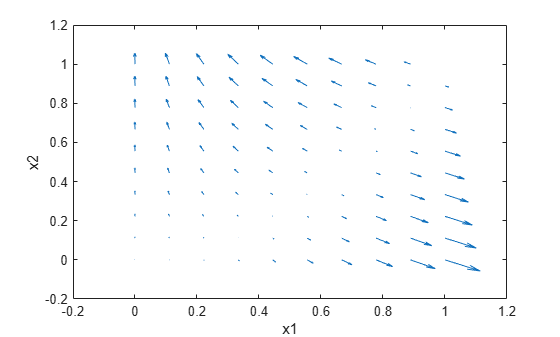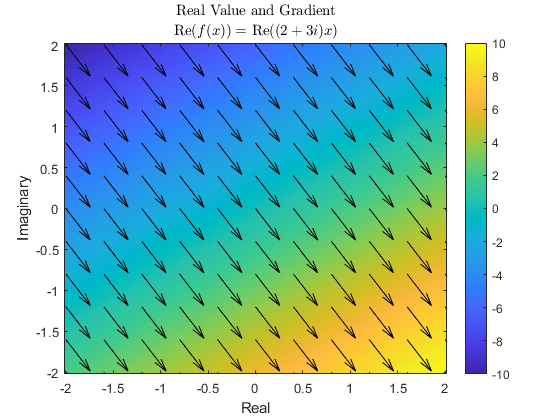# dlfeval

Evaluate deep learning model for custom training loops

## Syntax

``[y1,...,yk] = dlfeval(fun,x1,...,xn)``

## Description

Use `dlfeval` to evaluate custom deep learning models for custom training loops.

Tip

For most deep learning tasks, you can use a pretrained network and adapt it to your own data. For an example showing how to use transfer learning to retrain a convolutional neural network to classify a new set of images, see Train Deep Learning Network to Classify New Images. Alternatively, you can create and train networks from scratch using `layerGraph` objects with the `trainNetwork` and `trainingOptions` functions.

If the `trainingOptions` function does not provide the training options that you need for your task, then you can create a custom training loop using automatic differentiation. To learn more, see Define Deep Learning Network for Custom Training Loops.

example

````[y1,...,yk] = dlfeval(fun,x1,...,xn)` evaluates the deep learning array function `fun` at the input arguments `x1,...,xn`. Functions passed to `dlfeval` can contain calls to `dlgradient`, which compute gradients from the inputs `x1,...,xn` by using automatic differentiation.```

## Examples

collapse all

Rosenbrock's function is a standard test function for optimization. The `rosenbrock.m` helper function computes the function value and uses automatic differentiation to compute its gradient.

`type rosenbrock.m`
```function [y,dydx] = rosenbrock(x) y = 100*(x(2) - x(1).^2).^2 + (1 - x(1)).^2; dydx = dlgradient(y,x); end ```

To evaluate Rosenbrock's function and its gradient at the point `[–1,2]`, create a `dlarray` of the point and then call `dlfeval` on the function handle `@rosenbrock`.

```x0 = dlarray([-1,2]); [fval,gradval] = dlfeval(@rosenbrock,x0)```
```fval = 1x1 dlarray 104 ```
```gradval = 1x2 dlarray 396 200 ```

Alternatively, define Rosenbrock's function as a function of two inputs, `x1` and x`2`.

`type rosenbrock2.m`
```function [y,dydx1,dydx2] = rosenbrock2(x1,x2) y = 100*(x2 - x1.^2).^2 + (1 - x1).^2; [dydx1,dydx2] = dlgradient(y,x1,x2); end ```

Call `dlfeval` to evaluate `rosenbrock2` on two `dlarray` arguments representing the inputs `–1` and `2`.

```x1 = dlarray(-1); x2 = dlarray(2); [fval,dydx1,dydx2] = dlfeval(@rosenbrock2,x1,x2)```
```fval = 1x1 dlarray 104 ```
```dydx1 = 1x1 dlarray 396 ```
```dydx2 = 1x1 dlarray 200 ```

Plot the gradient of Rosenbrock's function for several points in the unit square. First, initialize the arrays representing the evaluation points and the output of the function.

```[X1 X2] = meshgrid(linspace(0,1,10)); X1 = dlarray(X1(:)); X2 = dlarray(X2(:)); Y = dlarray(zeros(size(X1))); DYDX1 = Y; DYDX2 = Y;```

Evaluate the function in a loop. Plot the result using `quiver`.

```for i = 1:length(X1) [Y(i),DYDX1(i),DYDX2(i)] = dlfeval(@rosenbrock2,X1(i),X2(i)); end quiver(extractdata(X1),extractdata(X2),extractdata(DYDX1),extractdata(DYDX2)) xlabel('x1') ylabel('x2')```Use `dlgradient` and `dlfeval` to compute the value and gradient of a function that involves complex numbers. You can compute complex gradients, or restrict the gradients to real numbers only.

Define the function `complexFun`, listed at the end of this example. This function implements the following complex formula:

`$\mathit{f}\left(\mathit{x}\right)=\left(2+3\mathit{i}\right)\mathit{x}$`

Define the function `gradFun`, listed at the end of this example. This function calls `complexFun` and uses `dlgradient` to calculate the gradient of the result with respect to the input. For automatic differentiation, the value to differentiate — i.e., the value of the function calculated from the input — must be a real scalar, so the function takes the sum of the real part of the result before calculating the gradient. The function returns the real part of the function value and the gradient, which can be complex.

Define the sample points over the complex plane between -2 and 2 and -2$\mathit{i}$ and 2$\mathit{i}$ and convert to `dlarray`.

```functionRes = linspace(-2,2,100); x = functionRes + 1i*functionRes.'; x = dlarray(x);```

Calculate the function value and gradient at each sample point.

```[y, grad] = dlfeval(@gradFun,x); y = extractdata(y);```

Define the sample points at which to display the gradient.

```gradientRes = linspace(-2,2,11); xGrad = gradientRes + 1i*gradientRes.';```

Extract the gradient values at these sample points.

```[~,gradPlot] = dlfeval(@gradFun,dlarray(xGrad)); gradPlot = extractdata(gradPlot);```

Plot the results. Use `imagesc` to show the value of the function over the complex plane. Use `quiver` to show the direction and magnitude of the gradient.

```imagesc([-2,2],[-2,2],y); axis xy colorbar hold on quiver(real(xGrad),imag(xGrad),real(gradPlot),imag(gradPlot),"k"); xlabel("Real") ylabel("Imaginary") title("Real Value and Gradient","Re\$(f(x)) = \$ Re\$((2+3i)x)\$","interpreter","latex")```The gradient of the function is the same across the entire complex plane. Extract the value of the gradient calculated by automatic differentiation.

`grad(1,1)`
```ans = 1×1 dlarray 2.0000 - 3.0000i ```

By inspection, the complex derivative of the function has the value

`$\frac{\mathrm{df}\left(\mathit{x}\right)}{\mathrm{dx}}=2+3\mathit{i}$`

However, the function Re($\mathit{f}\left(\mathit{x}\right)$) is not analytic, and therefore no complex derivative is defined. For automatic differentiation in MATLAB, the value to differentiate must always be real, and therefore the function can never be complex analytic. Instead, the derivative is computed such that the returned gradient points in the direction of steepest ascent, as seen in the plot. This is done by interpreting the function Re$\left(\mathit{f}\left(\mathit{x}\right)\right)$: C $\to$ R as a function Re$\left(\mathit{f}\left({\mathit{x}}_{\mathit{R}}+\mathit{i}{\mathit{x}}_{\mathit{I}}\right)\right)$: R $×$ R $\to$ R.

```function y = complexFun(x) y = (2+3i)*x; end function [y,grad] = gradFun(x) y = complexFun(x); y = real(y); grad = dlgradient(sum(y,"all"),x); end```

## Input Arguments

collapse all

Function to evaluate, specified as a function handle. If `fun` includes a `dlgradient` call, then `dlfeval` evaluates the gradient by using automatic differentiation. In this gradient evaluation, each argument of the `dlgradient` call must be a `dlarray` or a cell array, structure, or table containing a `dlarray`. The number of input arguments to `dlfeval` must be the same as the number of input arguments to `fun`.

Example: `@rosenbrock`

Data Types: `function_handle`

Function arguments, specified as any MATLAB data type or a `dlnetwork` object.

An input argument `xj` that is a variable of differentiation in a `dlgradient` call must be a traced `dlarray` or a cell array, structure, or table containing a traced `dlarray`. An extra variable such as a hyperparameter or constant data array does not have to be a `dlarray`.

To evaluate gradients for deep learning, you can provide a `dlnetwork` object as a function argument and evaluate the forward pass of the network inside `fun`.

Example: `dlarray([1 2;3 4])`

Data Types: `single` | `double` | `int8` | `int16` | `int32` | `int64` | `uint8` | `uint16` | `uint32` | `uint64` | `logical` | `char` | `string` | `struct` | `table` | `cell` | `function_handle` | `categorical` | `datetime` | `duration` | `calendarDuration` | `fi`
Complex Number Support: Yes

## Output Arguments

collapse all

Function outputs, returned as any data type. If the output results from a `dlgradient` call, the output is a `dlarray`.

## Version History

Introduced in R2019b